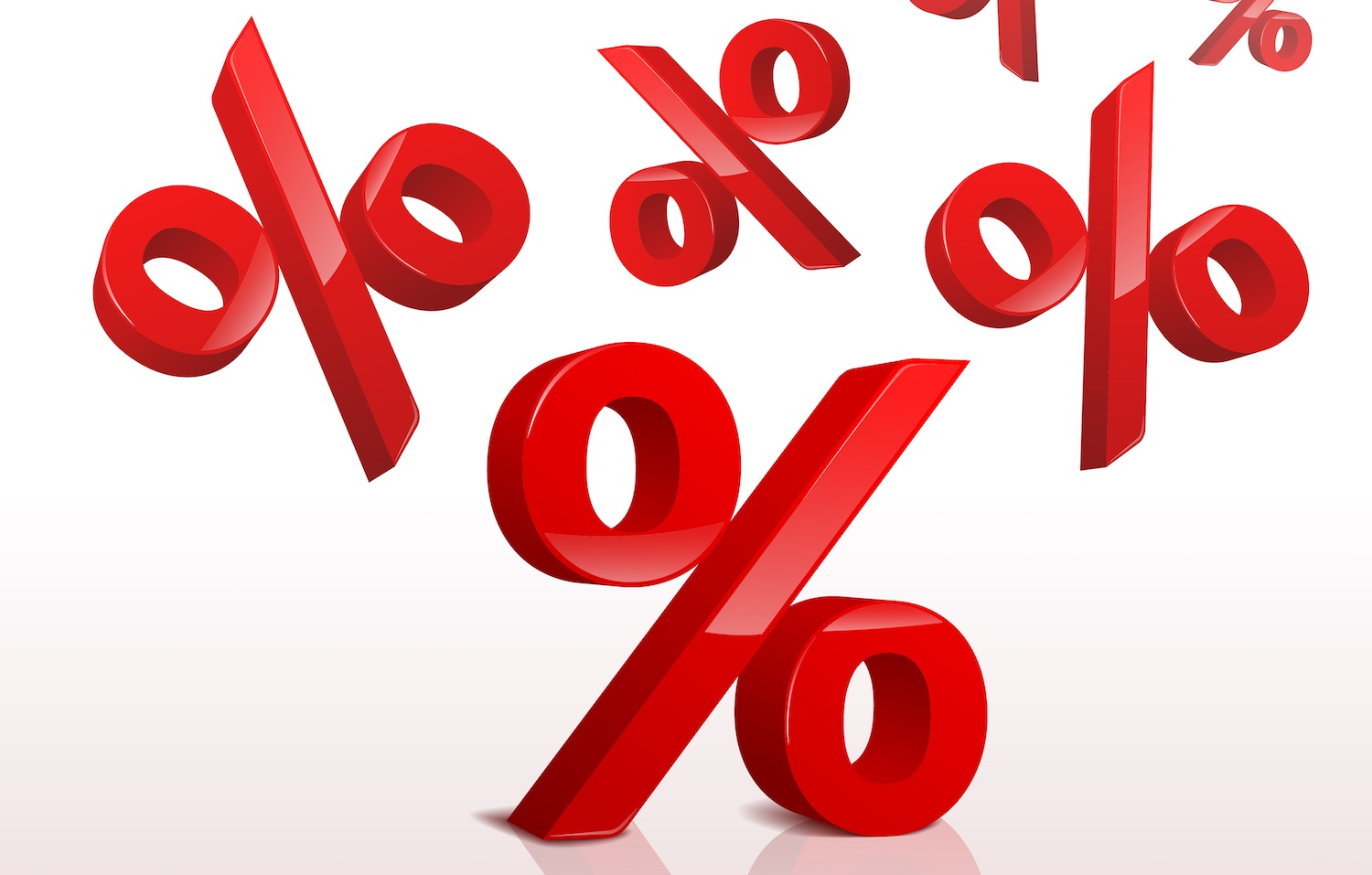# Percent of a Percent Calculator

Find the percentage of a percentage by entering them below. This is useful to find a compound percentage of a value.

%
%

## Result:

25% of 60% = 15%

### Steps to Solve

p=percent 1100×percent 2100×100
Substitute values in the equation
p=25100×60100×100
Simplify and solve
p=0.25×0.6×100
p=15%

You can also use our percentage calculator to find the portion of a value.

## How to Find the Percentage of a Percentage

Sometimes you need to find the percentage of a percentage, which might seem confusing. You can find the percent of a percent by converting both to decimal form, then multiplying them together.Start by dividing each percentage by 100 to convert them to a decimal form. Then multiply the decimals together to get the result. Multiply the result by 100 to convert the decimal back to a percentage.

### Percent of a Percent Formula

To find a percentage of a percentage, use the following formula:

p = pct1100 × pct2100 × 100

Thus the percentage of a percentage p is equal to the first percentage divided by 100 times the second percentage divided by 100, times 100.

Example: Find 25% of 80%

p = (pct1 ÷ 100) × (pct2 ÷ 100) × 100
p = (25 ÷ 100) × (80 ÷ 100) × 100
p = .25 × .8 × 100
p = .2 × 100
p = 20%

Check out our other percentage calculators for more tools for calculating percentages.